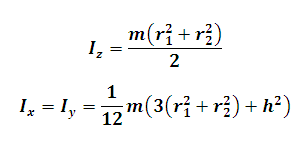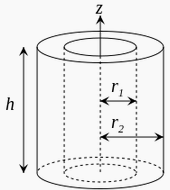# Moment of Inertia of a Thick Cylindrical Tube Calculator

This Calctown Calculator calculates the Moment of Inertia of a Thick Cylindrical Tube about a Perpendicular and Planar axis passing through the center of the cylinder.

kg
m
m
m

#### Result

kg-m2
kg-m2where

Iz = moment of inertia about perpendicular axis of rotation

Ix = Iy = moment of inertia about planar axis of rotation

m = mass of the cylinder

r1 = inner radius of the cylinder

r2 = outer radius of the cylinder

h = height of the cylinder